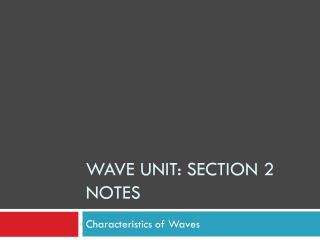DownloadDownload PresentationWave unit: Section 2 Notes

Wave unit: Section 2 Notes

Télécharger la présentationWave unit: Section 2 Notes

- - - - - - - - - - - - - - - - - - - - - - - - - - - E N D - - - - - - - - - - - - - - - - - - - - - - - - - - -
Presentation Transcript

1. Wave unit: Section 2 Notes Characteristics of Waves

2. Transverse Waves: • An ideal transverse wave has the shape of a sine curve and looks like an “S” lying on its side. • The simplest transverse waves have similar shapes no matter how big they are or what medium they travel through.

3. Parts of Transverse Waves • Crests: highest points of a transverse wave • Troughs: lowest points on a transverse wave • Amplitude: greatest distance that particles in a medium move from their normal position • Distance from the rest position to a crest or to a trough. • Wavelength: distance from one crest to the next or from one trough to the next. • Basically, it is the distance between any 2 successive identical parts of a wave.

4. Diagram of Transverse Waves crest crest trough trough

5. Parts of Longitudinal Waves • The particles move back & forth (parallel) instead of up & down (perpendicular) • Compressions: crowded areas in a longitudinal wave • Rarefactions: stretched out areas in a longitudinal wave • Wavelength: distance between 2 compressions or between 2 rarefactions

6. Diagram of a longitudinal wave:

7. Wavelength • In equations, wavelength is represented by the Greek letter lambda, λ • Because wavelength is a distance measurement, it is expressed in the SI unit meters.

8. Amplitude and Wavelength: Energy • The larger the amplitude of a wave, the more energy it carries. • Example: Waves of destructive earthquakes have greater amplitudes, and therefore more energy, than minor earthquakes. • The shorter the wavelength of a wave, the more energy it carries.

9. Period • Period: the time it takes one full wavelength of a wave to pass a certain point. • In equations, the period is represented by the symbol T. • Because period is a time measurement, it is expressed in the SI unit seconds (s).

10. Frequency • Frequency: the number of wavelengths that pass a point in a given time interval • The symbol for frequency is f. • SI Unit: Hertz (Hz) • Named after Heinrich Hertz, the scientist who demonstrated existence of electromagnetic waves in 1888. • Hertz units measure the number of vibrations per second. • We can hear sounds in the range from 20 Hz to 20,000 Hz.

11. Frequency-Period Equation:

12. Wave Speed

13. Wave Speed Example • The average wavelength in a series of ocean waves is 15.0 m. A wave crest arrives at the shore on average every 10.0 s, so the frequency is 0.100 Hz. What is the average speed of the waves? • v=f x λ • v= 0.100 Hz x 15.0 m • v= 1.50 m/s

14. Wave Speed and Medium • Speed of a wave depends on the medium: • Sound travels through air at 340 m/s (about 770 miles per hour) • Since sound travels fast in air, you don’t notice a time delay in most situations. • Sound travels three to four times fasterin water than in air. • If you swim with your head underwater, you can hear certain sounds very clearly. • Dolphins use sound waves to communicate with one another over long distances underwater.

15. Wave Speed and Medium • Sound waves travel even faster in solids than in air or water. • Sound waves have speeds 15 to 20 times as fast in rock or metal as in air. • Although the speed of a wave depends on the medium, the speed in a given medium is constant; it does not depend on the frequency of the wave. • No matter how fast you create waves on a rope, they still travel at the same speed; it just increases the frequency and decreases the wavelength.

16. Wave Speed and Phases of Matter • Wave speed in gases: a molecule must pass through a lot of empty space before it bumps into another molecule; therefore, waves don’t travel as fast in gases as they do in liquids and solids • Wave speed in liquids: particles are closer together & free to slide past one another & waves move faster • Wave speed in solids: particles are packed very tight together, so vibrations occur very rapidly,so waves travel very fast

17. Speed of Light • Speed of light in empty space: 3.0 x 108 m/s (671,000,000 mi/h) (186,000 mi/s) • The speed of light is a constant that is represented by the lowercase letter “c”. • Light travels slower when it has to pass through a medium like air or water.

18. The Doppler Effect • Have you ever been to a racetrack and noticed how the sound differs as the cars pass around the track? • The motion between the source of waves and the observer creates a change in observed frequency. In the case of sound waves, motion creates a change in pitch.

19. The Doppler Effect • The pitch, how high or low a sound is, is determined by the frequency of the waves. • A high-pitched sound is causedby sound waves of a higher frequency.

20. The Doppler Effect • A.) When an object is not moving, the frequency of the waves is the same at all locations. • B.) When an object is moving, the sound waves are closer in front and farther behind. The person in front hears a higher-pitched sound.

21. The Doppler Effect • So, the Doppler Effect is: an observed change in the frequency of a wave when the source or observer is moving.

22. The Doppler Effect